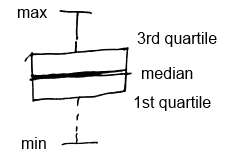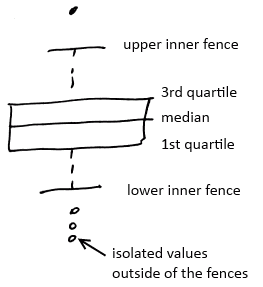# ML Wiki

## Five Number Summary

Consists of

• Minimum: the lowest point
• First quartile: the ¼ point in data
• Median: the center of data
• Third quartile: the ¾ point in dat.
• Maximum: the largest point

If a sample has even length, then the median is average of the two middle points:

• $\text{median} = \cfrac{a_{n/2} + a_{n/2 + 1}}{2}$

Same applies to 1st and 3rd quartiles

In R, command summary returns the 5 number summary as well as the mean

## Visualization

### Box Plot

A visual summary of all the 5 numbers is called a box plot

•Modified box plot

• is a variation of the box plot
• it's used to explain data with unusual values - Outliers
•## Measures of the Center

• Median is a measure of the center.
• But there is another measure - Mean or average value
$\text{mean} = \cfrac{1}{n} \sum x_i$
Where $n$ - number of data values, and $x_i$ - each data value.

Mean and median don't show how spread the data is. There is another measure that address it: Variance.

• $\text{var}(x) = \cfrac{1}{n - 1} \sum (x_i - \bar{x})^2$
• $s(x) = \text{std}(x) = \sqrt{\text{var}(x)}$
• ($n - 1$ gives "unbiased" estimate of the variance TODO: add link)

in R:

st.dev = sd(data)